Next: Approximate methods Up: Evaluation of uncertainty: general Previous: Indirect measurements   Contents

## Systematic errors

Uncertainty due to systematic effects is also included in a natural way in this approach. Let us first define the notation (is the generic index):
•is the n-tuple' (vector) of observables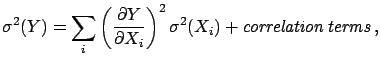;
•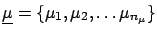is the n-tuple of true values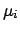;
•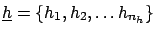is the n-tuple of influence quantities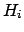.
By influence quantities we mean: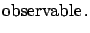all kinds of external factors which may influence the result (temperature, atmospheric pressure, etc.);all calibration constants;all possible hypotheses upon which the results may depend (e.g. Monte Carlo parameters).
From a probabilistic point of view, there is no distinction betweenand: they are all conditional hypotheses for the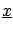, i.e. causes which produce the observed effects. The difference is simply that we are interested inrather than in2.17

There are alternative ways to take into account the systematic effects in the final distribution of:

1. Global inference on. We can use Bayes' theorem to make an inference onand, as described in Section: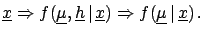This method, depending on the joint prior distribution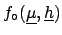, can even model possible correlations betweenand(e.g. radiative correction depending on the quantity of interest).
2. Conditional inference (see Fig.).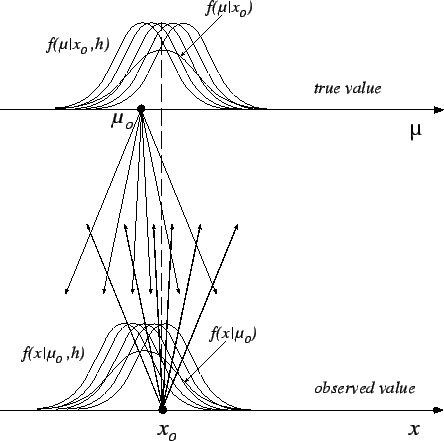Given the observed data, one has a joint distribution offor all possible configurations of: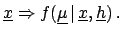Each conditional result is reweighed with the distribution of beliefs of, using the well-known law of probability: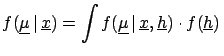d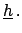(2.6)

3. Propagation of uncertainties. Essentially, one applies the propagation of uncertainty, whose most general case has been illustrated in the previous section, making use of the following model: One considers a raw result on raw values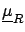for some nominal values of the influence quantities, i.e.then (corrected) true values are obtained as a function of the raw ones and of the possible values of the influence quantities, i.e.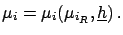The three ways lead to the same result and each of them can be more or less intuitive to different people, and more less suitable for different applications. For example, the last two, which are formally equivalent, are the most intuitive for HEP experimentalists, and it is conceptually equivalent to what they do when they vary -- within reasonable intervals -- all Monte Carlo parameters in order to estimate the systematic errors.2.18 The third form is particularly convenient to make linear expansions which lead to approximated solutions (see Section).

There is an important remark to be made. In some cases it is preferable not to integrate'2.19 over all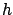's. Instead, it is better to report the result as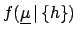, wherestands for a subset of, taken at their nominal values, if:

•could be controlled better by the users of the result (for example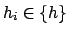is a theoretical quantity on which there is work in progress);
• there is some chance of achieving a better knowledge ofwithin the same experiment (for example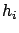could be the overall calibration constant of a calorimeter);
• a discrete and small number of very different hypotheses could affect the result. For example: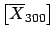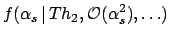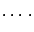This is, in fact, the standard way in which this kind of result has been presented (apart from the inessential fact that only best values and standard deviations are given, assuming normality).
If results are presented under the condition of, one should also report the derivatives ofwith respect to the result, so that one does not have to redo the complete analysis when the influence factors are better known. A typical example in which this is usually done is the possible variation of the result due to the precise values of the charm-quark mass. A recent example in which this idea has been applied thoroughly is given in Ref. .Next: Approximate methods Up: Evaluation of uncertainty: general Previous: Indirect measurements   Contents
Giulio D'Agostini 2003-05-15